The GLIMMIX Procedure
 LSMESTIMATE Statement
LSMESTIMATE fixed-effect <’label’> values <divisor=>
<, <’label’> values <divisor=>> <, ...>
< / options>
;
The LSMESTIMATE statement provides a mechanism for obtaining custom hypothesis tests among the least squares means. In contrast to the hypotheses tested with the ESTIMATE or CONTRAST statements, the LSMESTIMATE statement enables you to form linear combinations of the least squares means, rather than linear combination of fixed-effects parameter estimates and/or random-effects solutions. Multiple-row sets of coefficients are permitted.

The computation of an LSMESTIMATE involves two coefficient matrices. Suppose that the fixed-effect has
levels. Then the LS-means are formed as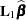, where
is a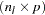coefficient matrix. The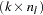coefficient matrix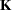is formed from the values that you supply in therows of the LSMESTIMATE statement. The least squares means estimates then represent the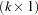vector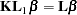The GLIMMIX procedure supports nonpositional syntax for the coefficients (values) in the LSMESTIMATE statement. For details see the section Positional and Nonpositional Syntax for Contrast Coefficients.

PROC GLIMMIX produces a t test for each row of coefficients specified in the LSMESTIMATE statement. You can adjust p-values and confidence intervals for multiplicity with the ADJUST= option. You can obtain an F test of single-row or multirow LSMESTIMATEs with the FTEST option.

Note that in contrast to a multirow estimate in the ESTIMATE statement, you specify only a single fixed effect in the LSMESTIMATE statement. The row labels are optional and follow the effects specification. For example, the following statements fit a split-split-plot design and compare the average of the third and fourth LS-mean of the whole-plot factor A to the first LS-mean of the factor:

```   proc glimmix;
class a b block;
model y = a b a*b /  s;
random int a / sub=block;
lsmestimate A 'a1 vs avg(a3,a4)' 2 0 -1 -1 divisor=2;
run;
```

The order in which coefficients are assigned to the least squares means corresponds to the order in which they are displayed in the "Least Squares Means" table. You can use the ELSM option to see how coefficients are matched to levels of the fixed-effect.

The optional divisor=n specification enables you to assign a separate divisor to each row of the LSMESTIMATE. You can also assign divisor values through the DIVISOR= option. See the documentation that follows for the interaction between the two ways of specifying divisors.

Many options of the LSMESTIMATE statement affect the computation of least squares means—for example, the AT=, BYLEVEL, and OM options. See the documentation for the LSMEANS statement for details.

You can specify the following options in the LSMESTIMATE statement after a slash (/).

specifies how denominator degrees of freedom are determined when p-values and confidence limits are adjusted for multiple comparisons with the ADJUST= option. When you do not specify the ADJDFE= option, or when you specify ADJDFE=SOURCE, the denominator degrees of freedom for multiplicity-adjusted results are the denominator degrees of freedom for the LS-mean effect in the "Type III Tests of Fixed Effects" table.

The ADJDFE=ROW setting is useful if you want multiplicity adjustments to take into account that denominator degrees of freedom are not constant across estimates. This can be the case, for example, when DDFM=SATTERTHWAITE or DDFM=KENWARDROGER is specified in the MODEL statement.

requests a multiple comparison adjustment for the p-values and confidence limits for the LS-mean estimates. The adjusted quantities are produced in addition to the unadjusted p-values and confidence limits. Adjusted confidence limits are produced if the CL or ALPHA= option is in effect. For a description of the adjustments, see Chapter 39, The GLM Procedure, and Chapter 58, The MULTTEST Procedure, of the SAS/STAT User’s Guide as well as the documentation for the ADJUST= option in the LSMEANS statement.

Note that not all adjustment methods of the LSMEANS statement are available for the LSMESTIMATE statement. Multiplicity adjustments in the LSMEANS statement are designed specifically for differences of least squares means.

If you specify the STEPDOWN option, the p-values are further adjusted in a step-down fashion.

ALPHA=number

requests that a t-type confidence interval be constructed for each of the LS-means with confidence level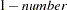. The value of number must be between 0 and 1; the default is 0.05.

AT variable=value
AT (variable-list)=(value-list)
AT MEANS

enables you to modify the values of the covariates used in computing LS-means. See the AT option in the LSMEANS statement for details.

BYLEVEL

requests that PROC GLIMMIX compute separate margins for each level of the LSMEANS effect.

The standard LS-means have equal coefficients across classification effects. The BYLEVEL option changes these coefficients to be proportional to the observed margins. This adjustment is reasonable when you want your inferences to apply to a population that is not necessarily balanced but has the margins observed in the input data set. In this case, the resulting LS-means are actually equal to raw means for fixed-effects models and certain balanced random-effects models, but their estimated standard errors account for the covariance structure that you have specified. If a WEIGHT statement is specified, PROC GLIMMIX uses weighted margins to construct the LS-means coefficients.

If the AT option is specified, the BYLEVEL option disables it.

CHISQ

requests that chi-square tests be performed in addition to F tests, when you request an F test with the FTEST option.

CL

requests that t-type confidence limits be constructed for each of the LS-means. If DDFM=NONE, then PROC GLIMMIX uses infinite degrees of freedom for this test, essentially computing a z interval. The confidence level is 0.95 by default; this can be changed with the ALPHA= option.

CORR

displays the estimated correlation matrix of the linear combination of the least squares means.

COV

displays the estimated covariance matrix of the linear combination of the least squares means.

DF=number

specifies the degrees of freedom for the t test and confidence limits. The default is the denominator degrees of freedom taken from the "Type III Tests of Fixed Effects" table corresponding to the LS-means effect.

DIVISOR=value-list

specifies a list of values by which to divide the coefficients so that fractional coefficients can be entered as integer numerators. If you do not specify value-list, a default value of 1.0 is assumed. Missing values in the value-list are converted to 1.0.

If the number of elements in value-list exceeds the number of rows of the estimate, the extra values are ignored. If the number of elements in value-list is less than the number of rows of the estimate, the last value in value-list is carried forward.

If you specify a row-specific divisor as part of the specification of the estimate row, this value multiplies the corresponding value in the value-list. For example, the following statement divides the coefficients in the first row by 8, and the coefficients in the third and fourth row by 3:

```   lsmestimate A 'One vs. two'   8 -8   divisor=2,
'One vs. three' 1  0 -1         ,
'One vs. four'  3  0  0 -3      ,
'One vs. five'  3  0  0  0  -3  / divisor=4,.,3;
```

Coefficients in the second row are not altered.

E

requests that the
coefficients of the estimable function be displayed. These are the coefficients that apply to the fixed-effect parameter estimates. The E option displays the coefficients that you would need to enter in an equivalent ESTIMATE statement.

ELSM

requests that thematrix coefficients be displayed. These are the coefficients that apply to the LS-means. This option is useful to ensure that you assigned the coefficients correctly to the LS-means.

EXP

requests exponentiation of the least squares means estimate. When you model data with the logit link function and the estimate represents a log odds ratio, the EXP option produces an odds ratio. See the section Odds and Odds Ratio Estimation for important details concerning the computation and interpretation of odds and odds ratio results with the GLIMMIX procedure. If you specify the CL or ALPHA= option, the (adjusted) confidence limits for the estimate are also exponentiated.

FTEST<(LABEL=’label’)>

produces an F test that jointly tests the rows of the LSMESTIMATE against zero. You can specify the optional label to identify the results from that test in the "LSMFtest" table.

requests that the estimate and its standard error also be reported on the scale of the mean (the inverse linked scale). PROC GLIMMIX computes the value on the mean scale by applying the inverse link to the estimate. The interpretation of this quantity depends on the coefficients specified in your LSMESTIMATE statement and the link function. In a model for binary data with logit link, for example, the following LSMESTIMATE statement computes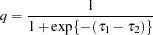where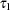and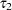are the least squares means associated with the first two levels of the classification effect A:

```   proc glimmix;
class A;
model y = A / dist=binary link=logit;
lsmestimate A 1 -1 / ilink;
run;
```

The quantityis not the difference of the probabilities associated with the two levels,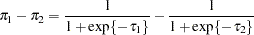The standard error of the inversely linked estimate is based on the delta method. If you also specify the CL or ALPHA= option, the GLIMMIX procedure computes confidence intervals for the inversely linked estimate. These intervals are obtained by applying the inverse link to the confidence intervals on the linked scale.

LOWER
LOWERTAILED

requests that the p-value for the t test be based only on values less than the test statistic. A two-tailed test is the default. A lower-tailed confidence limit is also produced if you specify the CL or ALPHA= option.

Note that for ADJUST=SCHEFFE the one-sided adjusted confidence intervals and one-sided adjusted p-values are the same as the corresponding two-sided statistics, because this adjustment is based on only the right tail of the F distribution.

OBSMARGINS
OM

specifies a potentially different weighting scheme for the computation of LS-means coefficients. The standard LS-means have equal coefficients across classification effects; however, the OM option changes these coefficients to be proportional to those found in the input data set. See the OBSMARGINS option in the LSMEANS statement for further details.

SINGULAR=number

tunes the estimability checking as documented for the CONTRAST statement.

STEPDOWN<(step-down-options)>

requests that multiplicity adjustments for the p-values of LS-mean estimates be further adjusted in a step-down fashion. Step-down methods increase the power of multiple testing procedures by taking advantage of the fact that a p-value will never be declared significant unless all smaller p-values are also declared significant. Note that the STEPDOWN adjustment combined with ADJUST=BON corresponds to the methods of Holm (1979) and Shaffer’s "Method 2" (1986); this is the default. Using step-down-adjusted p-values combined with ADJUST=SIMULATE corresponds to the method of Westfall (1997).

If the degrees-of-freedom method is DDFM=KENWARDROGER or DDFM=SATTERTHWAITE, then step-down-adjusted p-values are produced only if the ADJDFE=ROW option is in effect.

Also, the STEPDOWN option affects only p-values, not confidence limits. For ADJUST=SIMULATE, the generalized least squares hybrid approach of Westfall (1997) is employed to increase Monte Carlo accuracy.

You can specify the following step-down-options in parentheses:

MAXTIME=n

specifies the time (in seconds) to spend computing the maximal logically consistent sequential subsets of equality hypotheses for TYPE=LOGICAL. The default is MAXTIME=60. If the MAXTIME value is exceeded, the adjusted tests are not computed. When this occurs, you can try increasing the MAXTIME value. However, note that there are common multiple comparisons problems for which this computation requires a huge amount of time—for example, all pairwise comparisons between more than 10 groups. In such cases, try to use TYPE=FREE (the default) or TYPE=LOGICAL() for small.

ORDER=PVALUE
ORDER=ROWS

specifies the order in which the step-down tests are performed. ORDER=PVALUE is the default, with LS-mean estimates being declared significant only if all LS-mean estimates with smaller (unadjusted) p-values are significant. If you specify ORDER=ROWS, then significances are evaluated in the order in which they are specified.

REPORT

specifies that a report on the step-down adjustment be displayed, including a listing of the sequential subsets (Westfall 1997) and, for ADJUST=SIMULATE, the step-down simulation results.

TYPE=LOGICAL<(n)>
TYPE=FREE

If you specify TYPE=LOGICAL, the step-down adjustments are computed by using maximal logically consistent sequential subsets of equality hypotheses (Shaffer 1986, Westfall 1997). Alternatively, for TYPE=FREE, sequential subsets are computed ignoring logical constraints. The TYPE=FREE results are more conservative than those for TYPE=LOGICAL, but they can be much more efficient to produce for many estimates. For example, it is not feasible to take logical constraints between all pairwise comparisons of more than about 10 groups. For this reason, TYPE=FREE is the default.

However, you can reduce the computational complexity of taking logical constraints into account by limiting the depth of the search tree used to compute them, specifying the optional depth parameter as a numberin parentheses after TYPE=LOGICAL. As with TYPE=FREE, results for TYPE=LOGICAL() are conservative relative to the true TYPE=LOGICAL results, but even for TYPE=LOGICAL(), they can be appreciably less conservative than TYPE=FREE, and they are computationally feasible for much larger numbers of estimates. If you do not specifyor if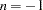, the full search tree is used.

UPPER
UPPERTAILED

requests that the p-value for the t test be based only on values greater than the test statistic. A two-tailed test is the default. An upper-tailed confidence limit is also produced if you specify the CL or ALPHA= option.

Note that for ADJUST=SCHEFFE the one-sided adjusted confidence intervals and one-sided adjusted p-values are the same as the corresponding two-sided statistics, because this adjustment is based on only the right tail of the F distribution.Previous Page | Next Page | Top of Page